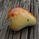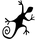23703瀏覽
This is my custom channel (Bear Channel!? :)) derived from BB/STARC. It uses both ATR/STDEV for plotting the bounds.

I use COG (Center of Gravity ) for deriving the baseline. This enables it to track the price action better than many other channels that make use of MAs or simply "close". Indicator also marks "squeezes" (stdev bands come inside ATR bands). Pay attention to these, as these usually indicate a move.

I am still exploring this indicator on different BTCUSD time frames, would love to hear your feedback / setups for other instruments.

Code for this indicator: http://pastebin.com/QXBJqAhA

Code for COG fibs I mentioned in the comments: http://pastebin.com/CbxY31at
```//
// @author LazyBear
//
// This is my custom channel derived from BB/STARC. This uses both ATR/STDEV for plotting the bounds.
// I use COG for the base line (normally it is SMA/EMA).
//
// If you use this code in its original/modified form, do drop me a note.
//
study("COG Double Channel [LazyBear]", shorttitle="COGChannel_LB", overlay=true)
src = close
length = input(34)
median=0
mult=input(2.5)
offset = input(20)
tr_custom() =>
x1=high-low
x2=abs(high-close)
x3=abs(low-close)
max(x1, max(x2,x3))

atr_custom(x,y) =>
sma(x,y)

dev = (mult * stdev(src, length))
basis=linreg(src, length, median)
ul = (basis + dev)
ll = (basis - dev)
tr_v = tr_custom()
acustom=(2*atr_custom(tr_v, length))
uls=basis+acustom
lls=basis-acustom

// Plot STDEV channel
plot(basis, linewidth=1, color=navy, style=line, linewidth=1, title="Median")
lb=plot(ul, color=red, linewidth=1, title="BB+", style=dashed)
tb=plot(ll, color=green, linewidth=1, title="BB-", style=dashed)
fill(tb,lb, silver, title="Region fill")

// Plot ATR channel
plot(basis, linewidth=2, color=navy, style=line, linewidth=2, title="Median")
ls=plot(uls, color=red, linewidth=1, title="Starc+", style=circles)
ts=plot(lls, color=green, linewidth=1, title="Star-", style=circles)
fill(ts,tb, green, title="Region fill")
fill(ls,lb, red, title="Region fill")

// Mark SQZ
plot_offs_high=2
plot_offs_low=2
sqz_f=(uls>ul) and (lls<ll)
b_color=sqz_f ? teal : na
plot(sqz_f ? lls-plot_offs_low : na, color=b_color, style=cross, linewidth=2)
plot(sqz_f ? uls+plot_offs_high : na, color=b_color, style=cross, linewidth=2)
```
List of my free indicators: http://bit.ly/1LQaPK8
List of my indicators at Appstore: http://blog.tradingview.com/?p=970

## 評論KurbelklausQuick visual comparison of this channel with BB. Similar ATR comparison can be made too.Applied your indicator to NVIDA, it says a move is coming . I agree. Thank you !!littleriver
Nice. BTW, you may want to use the code I have given in the link -- http://pastebin.com/QXBJqAhA <-- this has the suggested changes from @TheLark.What about a fibo bear channel like this one ;)magarto
A similar Fib channel, based on COGs though